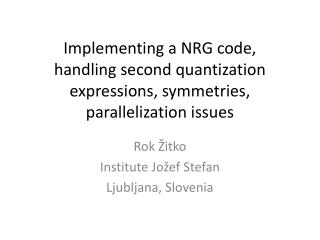Download PresentationRok Žitko Institute Jožef Stefan Ljubljana, Slovenia

# Rok Žitko Institute Jožef Stefan Ljubljana, Slovenia

Download Presentation## Rok Žitko Institute Jožef Stefan Ljubljana, Slovenia

- - - - - - - - - - - - - - - - - - - - - - - - - - - E N D - - - - - - - - - - - - - - - - - - - - - - - - - - -
##### Presentation Transcript

1. Implementing a NRG code, handling second quantization expressions, symmetries,parallelization issues Rok Žitko Institute Jožef Stefan Ljubljana, Slovenia

2. Tools: SNEG and NRG Ljubljana Add-on package for the computer algebra system Mathematica for performing calculations involving non-commuting operators • Efficient general purpose numerical renormalization group code • flexible and adaptable • highly optimized (partially parallelized) • easy to use

3. t e, U e, U

4. SNEG - features • fermionic (Majorana, Dirac) and bosonic operators, Grassman numbers • basis construction (well defined number and spin (Q,S), isospin and spin (I,S), etc.) • symbolic sums over dummy indexes (k, s) • Wick’s theorem (with either empty band or Fermi sea vacuum states) • Dirac’s bra and ket notation • Simplifications using Baker-Campbell-Hausdorff and Mendaš-Milutinović formula

5. SNEG - applications • exact diagonalization of small clusters • perturbation theory to high order • high-temperature series expansion • evaluation of (anti-)commutators of complex expressions • NRG • derivation of coefficients required in the NRG iteration • problem setup

6. “NRG Ljubljana” - goals • Flexibility (very few hard-coded limits, adaptability) • Implementation using modern high-level programming paradigms (functional programming in Mathematica, object oriented programming in C++)  short and maintainable code • Efficiency (LAPACK routines for diagonalization) • Free availability

7. f0,L f0,R a b Definition of a quantum impurity problem in “NRG Ljubljana” t Himp = eps (number[a[]]+number[b[]])+U/2 (pow[number[a[]]-1,2]+pow[number[b[]]-1,2]) Hab = t hop[a[],b[]] Hc = Sqrt[Gamma] (hop[a[],f[L]] + hop[b[],f[R]]) + J spinspin[a[],b[]] + V chargecharge[a[],b[]]

8. f0,L f0,R a b Definition of a quantum impurity problem in “NRG Ljubljana” t Himp = epsa number[a[]] + epsb number[b[]] +U/2 (pow[number[a[]]-1,2]+pow[number[b[]]-1,2]) Hab = t hop[a[],b[]] Hc = Sqrt[Gamma] (hop[a[],f[L]] + hop[b[],f[R]])

9. “By relieving the brain of all unnecessary work, a good notation sets it free to concentrate on more advancedproblems, and in effect increases the mental power of the race.” Alfred North Whitehead

10. nrginit nrgrun various scripts

11. Computable quantities • Finite-site excitation spectra (flow diagrams) • Thermodynamics: magnetic and charge susceptibility, entropy, heat capacity • Correlations: spin-spin correlations, charge fluctuations,...spinspin[a[],b[]] number[d[]]pow[number[d[]], 2] • Dynamics: spectral functions, dynamical magnetic and charge susceptibility, other response functions

12. Spectral function Charge fluctuations Occupancy Sample input file [param]model=SIAMU=1.0Gamma=0.04 Lambda=3Nmax=40keepenergy=10.0keep=2000 ops=q_d q_d^2 A_d Model and parameters NRG iteration parameters Computed quantities

13. Spin symmetry

14. Charge and particle-hole symmetry

15. Isospin symmetry Nambu spinor: Izospinoperator: charge pairing

16. Reflection symmetry Parity Z2 quantum number P "Flavor symmetry" SU(2)flavor:

17. Wigner-Eckart theorem O is a spherical tensor operator of rank M if: Clebsch-Gordan coefficients for SU(2) For a more general treatment of non-Abelian symmetries in NRG, see A. I. Toth, C. P. Moca, O. Legeza, G. Zarand, PRB 78, 245109 (2008), A. Weichselbaum, Annals of Physics 327, 2972-3047 (2012).

18. Diagonalization • Full diagonalizations with dsyev/zheev • Partial diagonalizations with dsyevr/zheevr(possible when CFS/FDM is not used) • For most problems this is where the largest amount of the processor time is spent. • Note: symmetric eigenvalue problem has a high memory to arithmetic ratio. Unclear if GPUs would help much for large problems.

19. Recalculation of operators Important to be efficiently implemented! We use BLAS routine GEMM (general matrix multiply). (GEMM from Intel MKL library has >80% efficiency on Xeon processors.)

20. Parallelization • Multi-threading on multi-processor computers (pthreads or OpenMP). • Intel MKL implementation of LAPACK takes advantage of multi-core CPUs. • DSYEV does not scale linearly, but there is some speedup. • Parallelization across multiple computers using message passing (MPI). • Parallel diagonalisations using LAPACK, or parallelized diagonalisation using ScaLAPACK.

21. Matrix dimensions in different invariant subspaces. SIAM, U(1)charge x U(1)spin symmetry type

22. Conclusion: up to ~5-6 simultaneous diagonalisations.

23. Master-slave strategy using MPI • Master delegates diagonalisations of large matrices to slave nodes. • Master diagonalizes small matrices locally. master slaves Communication overhead is negligible!

24. OpenMP + MPI • Best so far: spread calculation across 5-6 nodes, use multi-threaded DSYEV on each node (4 threads). • More recently: 4 CPUs, 8 threads each. • TO DO: evaluate ScaLAPACK on machines with fast interconnect (such as Infiniband).

25. Nested parallelism with OpenMP & Intel MKL OMP_NESTED=TRUE OMP_NUM_THREADS=4 MKL_NUM_THREADS=16 MKL_DYNAMIC=FALSE Significant improvement, when it works! (Segmentation faults,...)

26. Toy implementation of NRG • http://nrgljubljana.ijs.si/nrg.nb • Implements SIAM in (Q,S) basis, it computes flow diagrams,thermodynamics and expectation values • Reasonably fast (Mathematica internally uses Intel MKL libraries for numerical linear algebra and there is little overhead)